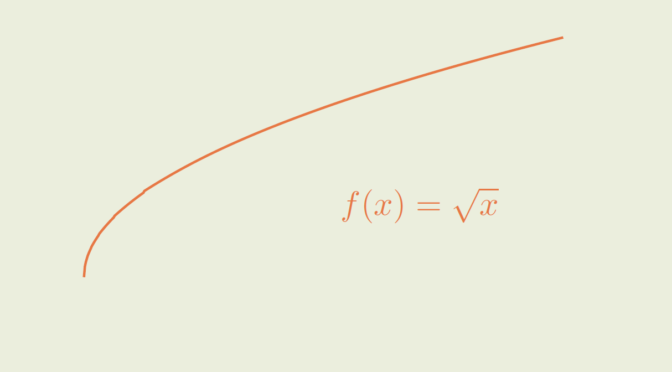# Uniform continuous function but not Lipschitz continuous

Consider the function $\begin{array}{l|rcl} f : & [0,1] & \longrightarrow & [0,1] \\ & x & \longmapsto & \sqrt{x} \end{array}$

$$f$$ is continuous on the compact interval $$[0,1]$$. Hence $$f$$ is uniform continuous on that interval according to Heine-Cantor theorem. For a direct proof, one can verify that for $$\epsilon > 0$$, one have $$\vert \sqrt{x} – \sqrt{y} \vert \le \epsilon$$ for $$\vert x – y \vert \le \epsilon^2$$.

However $$f$$ is not Lipschitz continuous. If $$f$$ was Lipschitz continuous for a Lipschitz constant $$K > 0$$, we would have $$\vert \sqrt{x} – \sqrt{y} \vert \le K \vert x – y \vert$$ for all $$x,y \in [0,1]$$. But we get a contradiction taking $$x=0$$ and $$y=\frac{1}{4 K^2}$$ as $\vert \sqrt{x} – \sqrt{y} \vert = \frac{1}{2 K} > \frac{1}{4 K} = K \vert x – y \vert$